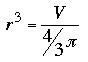SEARCH HOMEMath Central Quandaries & QueriesQuestion from Cey, a student: how to i find the radius of a sphere with a volume of 1000cm cubed using the formula v=4/3 pi r squared???????/Cey,

The volume of a sphere is

V = 4/3 π r3 not squared.

To find the radius if you know the volume, divide both sides of the equation above to getEvaluate the right side and then take the cube root to find r.

PennyMath Central is supported by the University of Regina and The Pacific Institute for the Mathematical Sciences.